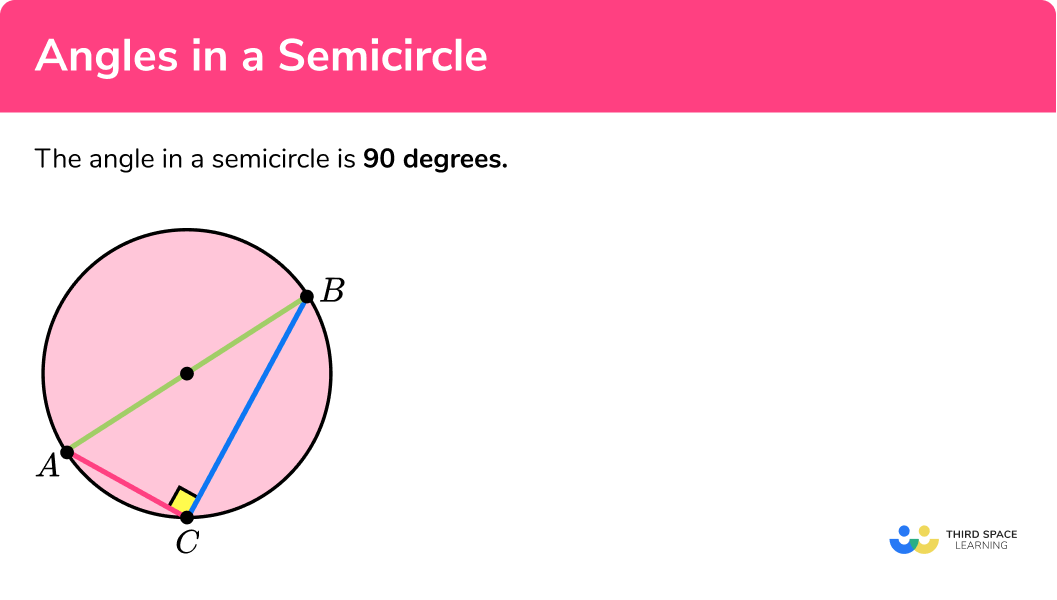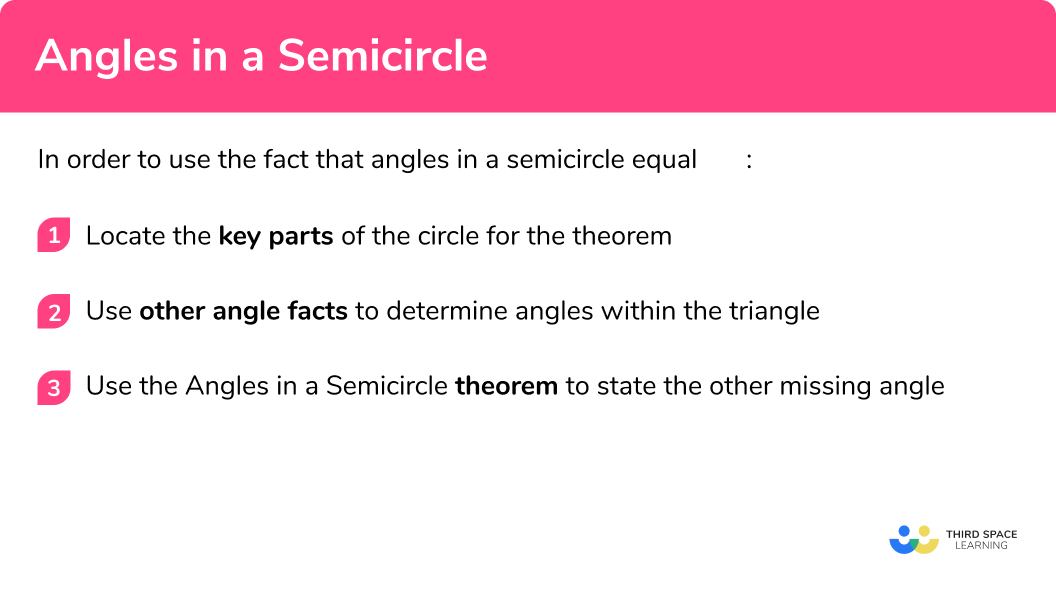# Angle In A Semicircle

Here we will learn about angles in a semicircle, including their application, proof, and using them to solve more difficult problems.

There are also circle theorem worksheets based on Edexcel, AQA and OCR exam questions, along with further guidance on where to go next if you’re still stuck.

## What are angles in a semicircle?

Angles in a semicircle are 90 degrees:

Angles in a semicircle are created when you join the two ends of the diameter to one point on the arc using chords.

In the diagram above, AB is the diameter of a circle that divides the circle into two. The two points A and B are joined to another point C on the circumference using two chords. This triangle is a right-angle triangle with the 90 degree angle touching the arc. This is the same for any point that is placed on the arc.

If the point is placed on another point in a semicircle, the result would be the same (the two chords would meet at the circumference at \pmb{90} degrees). The angle in a semicircle is 90 degrees.

### What are angles in a semicircle?### Key parts of a circle needed for this theorem

• The diameter of the circle is the width of a circle, through the centre. The diameter is twice the length of the radius of a circle. In a semicircle, the diameter is the straight edge.
• A chord is a straight line that meets the circumference in two places. The longest chord in a circle is the diameter.
• A semicircle is half of a circle.

Subtended angles (in a semicircle):

An angle within a semicircle is created by two chords meeting at the two ends of the diameter. The diagrams below show the angle subtended by diameter AC from point B for two different semicircles:

### Proving that angles in the same segment are equal

To be able to prove this theorem, you do not need to know any other circle theorem. You just need to be confident with angles in a triangle.

## How to use the angle in a semicircle theorem

In order to use the fact that angles in a semicircle equal 90° :

1. Locate the key parts of the circle for the theorem.
2. Use other angle facts to determine angles within the triangle.
3. Use the angles in a semicircle theorem to state the other missing angle.

### How to use the angle in a semicircle theoremTangent of a circle is one of 7 circle theorems you will need to know. You may find it helpful to start with our main circle theorems page and then look in detail at the rest.

## Angle in a semicircle examples

### Example 1: standard diagram

ABCD is an arrowhead where C is the centre of the circle and A, B, and D lie on the circumference. Calculate the size of angle BAD :

1. Locate the key parts of the circle for the theorem.

Here we have:

• The angle ABC = 67°
• AB is a diameter
• AC and BC are chords
• The angle CAB = θ

2Use other angle facts to determine angles within the triangle.

We already know that ABC=67° so we do not need to use any other angle fact to determine this angle for this example.

3Use the angles in a semicircle theorem to state the other missing angle.

As the angle in a semicircle is equal to 90° , angle ACB = 90° . We can therefore use the fact that angles in a triangle total 180° to calculate the size of angle BAC :

BAC = 180 - (90 + 67)

BAC=23°

ABCD is a cyclic quadrilateral. ACD is an isosceles triangle. Calculate the size of angle ACB .

Locate the key parts of the circle for the theorem.

Use other angle facts to determine angles within the triangle.

Use the angles in a semicircle theorem to state the other missing angle.

### Example 3: angles in the same segment

Points A, B, C, and D lie on the circumference of the circle. AD is a diameter of the circle. BD and AC intersect at the point E . Calculate the size of the angle CDB .

Locate the key parts of the circle for the theorem.

Use other angle facts to determine angles within the triangle.

Use the angles in a semicircle theorem to state the other missing angle.

### Example 4: angles in parallel lines

AC and BD are diameters of the circle with centre O . BC and AD are parallel. Calculate the size of the angle OAB .

Locate the key parts of the circle for the theorem.

Use other angle facts to determine angles within the triangle.

Use the angles in a semicircle theorem to state the other missing angle.

### Example 5: the alternate segment theorem

ABCD are points on a circle with centre O . EF is a tangent to the circle through the point C . BD and EF are parallel. Angle DCE = 63° .

Calculate the size of angle BAC .

Locate the key parts of the circle for the theorem.

Use other angle facts to determine angles within the triangle.

Use the angles in a semicircle theorem to state the other missing angle.

### Example 6: angle at the centre

A, B, C, D and E are points on the circle with centre O . Points A and O lie on a line of symmetry. Calculate the size of the angle DAE .

Locate the key parts of the circle for the theorem.

Use other angle facts to determine angles within the triangle.

Use the angles in a semicircle theorem to state the other missing angle.

### Common misconceptions

The angles that are either end of the diameter total 180° as if the triangle were a cyclic quadrilateral. They should total 90° as the angle in a semicircle is 90° .

• Isosceles triangles

The triangle is assumed to be isosceles and so the angles at either end of the diameter are the same.

• A diameter or a chord?

The angle at the circumference is assumed to be 90° when the associated chord does not intersect the centre of the circle and so the diagram does not show a semicircle.

• Incorrect angle

The angle that is established as 90° , is labelled as an angle by the diameter, and not the subtended angle at the circumference.

### Practice angle in a semicircle questions

1. The points A, B, and C are on the circumference of a circle. Calculate the size of angle BCA .127^{\circ}37^{\circ}53^{\circ}90^{\circ}• ABC = 90^{\circ} (angles in a semicircle)
• BCA = 180 – (90+53) = 37^{\circ}

2. The points A, B, and C are on the circumference of a circle. Angle ABC = 79^{\circ}. Calculate the size of angle BCA .56^{\circ}101^{\circ}79^{\circ}50.5^{\circ}• BAC = BCD = 90^{\circ} (angles in a semicircle)
• ABC = (180 – 90)\div2 = 45^{\circ} (isosceles triangle)
• CBD = 79 – 45 = 34^{\circ}
• CDB = 180 – (90+34) = 56^{\circ}

3. The points A, B, C, and D are on the circumference of a circle. AC and BD are chords that intersect at the point E . Calculate the size of angle DAE .63^{\circ}35^{\circ}55^{\circ}27^{\circ}• AED = 35^{\circ} (vertically opposite angles are equal)
• ADE = 90^{\circ} (angles in a semicircle)
• DAE = 180 – (90+35)=55^{\circ}

4. The points A, B, C, and D are points on the circumference of a circle. AC and BC intersect at the centre O at an angle of 152^{\circ} . Calculate the size of angle ABO .76^{\circ}28^{\circ}14^{\circ}90^{\circ}• BOC = 152^{\circ} (vertically opposite angles are equal)
• OBC = (180-152)\div2 = 14^{\circ} (isosceles triangle)
• ABO = 90 – 14 = 76^{\circ}

5. The points A, B, C and D are on the circumference of a circle with centre O . The chords AC and BD intersect at the point G . AC is parallel to the tangent EF which passes through the point B . Angle FBC = 70^{\circ} . Calculate the size of angle BDC .90^{\circ}40^{\circ}20^{\circ}70^{\circ}• CBG = 90 – 70 = 20^{\circ} (tangent meets radius at 90^{\circ} )
• BCD = 90^{\circ} (angles in a semicircle)
• BDC = 180 – (90+20) = 70^{\circ} (angles in a triangle)

6. The points A, B, C, D and E are on the circumference of a circle with centre O . AB = AE , and AD = AC. Angle ACD = 26^{\circ} . Calculate the size of angle DAE .24^{\circ}42^{\circ}21^{\circ}45^{\circ}• CAD = 180 – (66+66) = 48^{\circ} (isosceles triangle)
• BAE = 90^{\circ} (angles in a semicircle)
• DAE = (90-48)\div2 = 21^{\circ}

### Angle in a semicircle GCSE questions

1.

(a) The circle with centre O has a diameter AD . Points A, B, C, and D lie on the circumference of the circle and AC intersects with BD at the point E . Calculate the size of angle ABE marked x . Show your working.(b) Hence calculate the size of angle BAE , marked y .(10 marks)

(a)

DCE = 90^{\circ}

(1)

The angle in a semicircle is 90^{\circ}

(1)

DEC = 180 – (90+40) = 50^{\circ}

(1)

Angles in a triangle

(1)

BEA = 50^{\circ}

(1)

Vertically opposite angles are equal

(1)

(b)

ABE = 90^{\circ}

(1)

The angle in a semicircle is 90^{\circ}

(1)

BAE = 180 – (90+50) = 40^{\circ}

(1)

Angles in a triangle

(1)

2. ABC is a triangle inscribed into a circle. Calculate the size of angle ABC .(4 marks)

BAC = 90^{\circ}

(1)

The angle in a semicircle is 90^{\circ}

(1)

ABC = 180 – (90 + 15) = 75^{\circ}

(1)

Angles in a triangle total 180^{\circ}

(1)

3.

(a) By calculating the size of angle ACD , find the size of angle \theta . State any reasons with your answer.(b) What type of triangle is BCD ?

(5 marks)

(a)

ACD = (180 – 120)\div2 = 30^{\circ}

(1)

ACB = 90^{\circ}

(1)

The angle in a semicircle is 90^{\circ}

(1)

BCD = 90 – 30 = 60^{\circ}

(1)

(b)

Equilateral

(1)

## Learning checklist

You have now learned how to:

• Apply and prove the standard circle theorems concerning angles, radii, tangents and chords, and use them to prove related results

## Still stuck?

Prepare your KS4 students for maths GCSEs success with Third Space Learning. Weekly online one to one GCSE maths revision lessons delivered by expert maths tutors.

Find out more about our GCSE maths tuition programme.